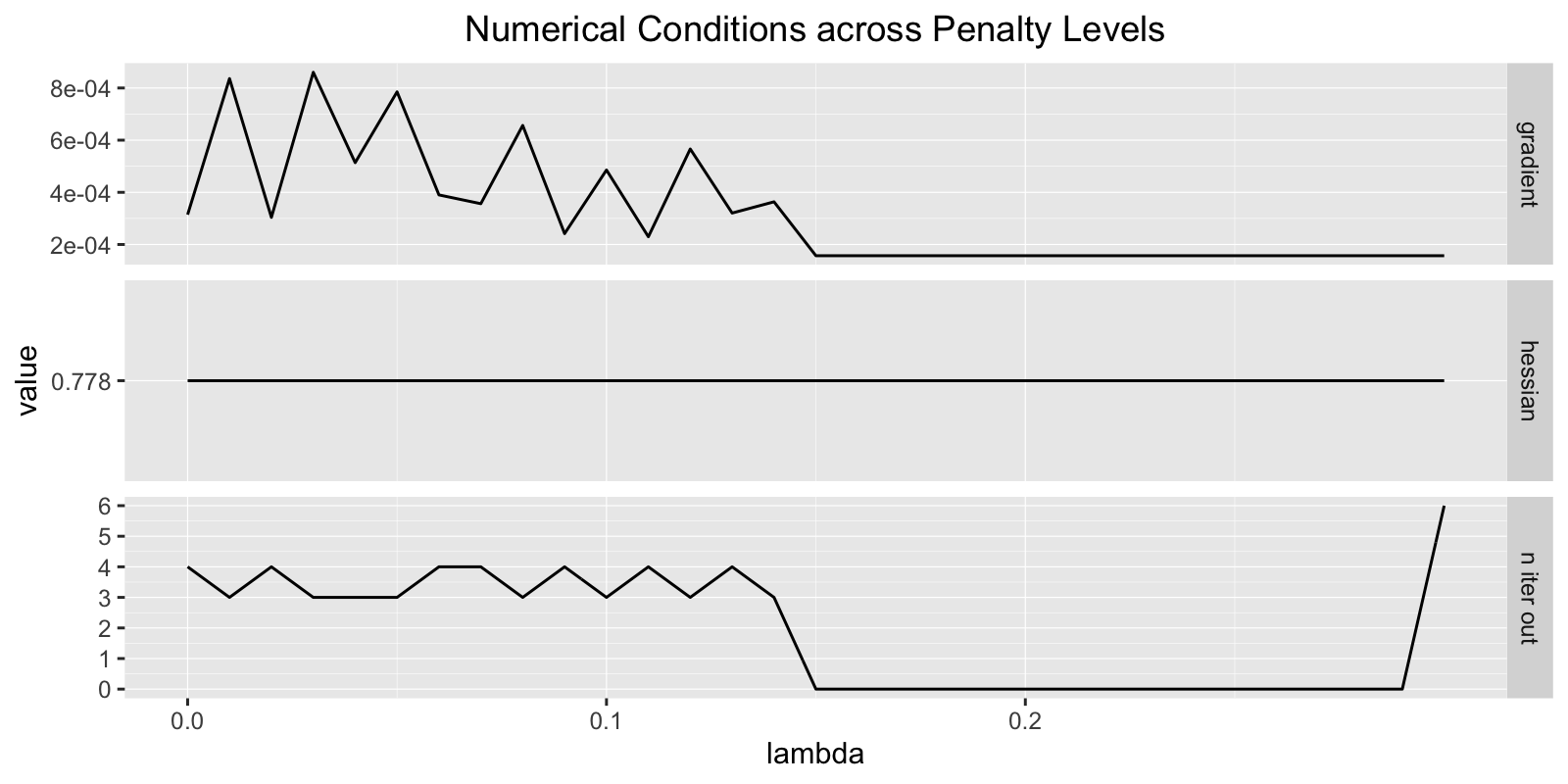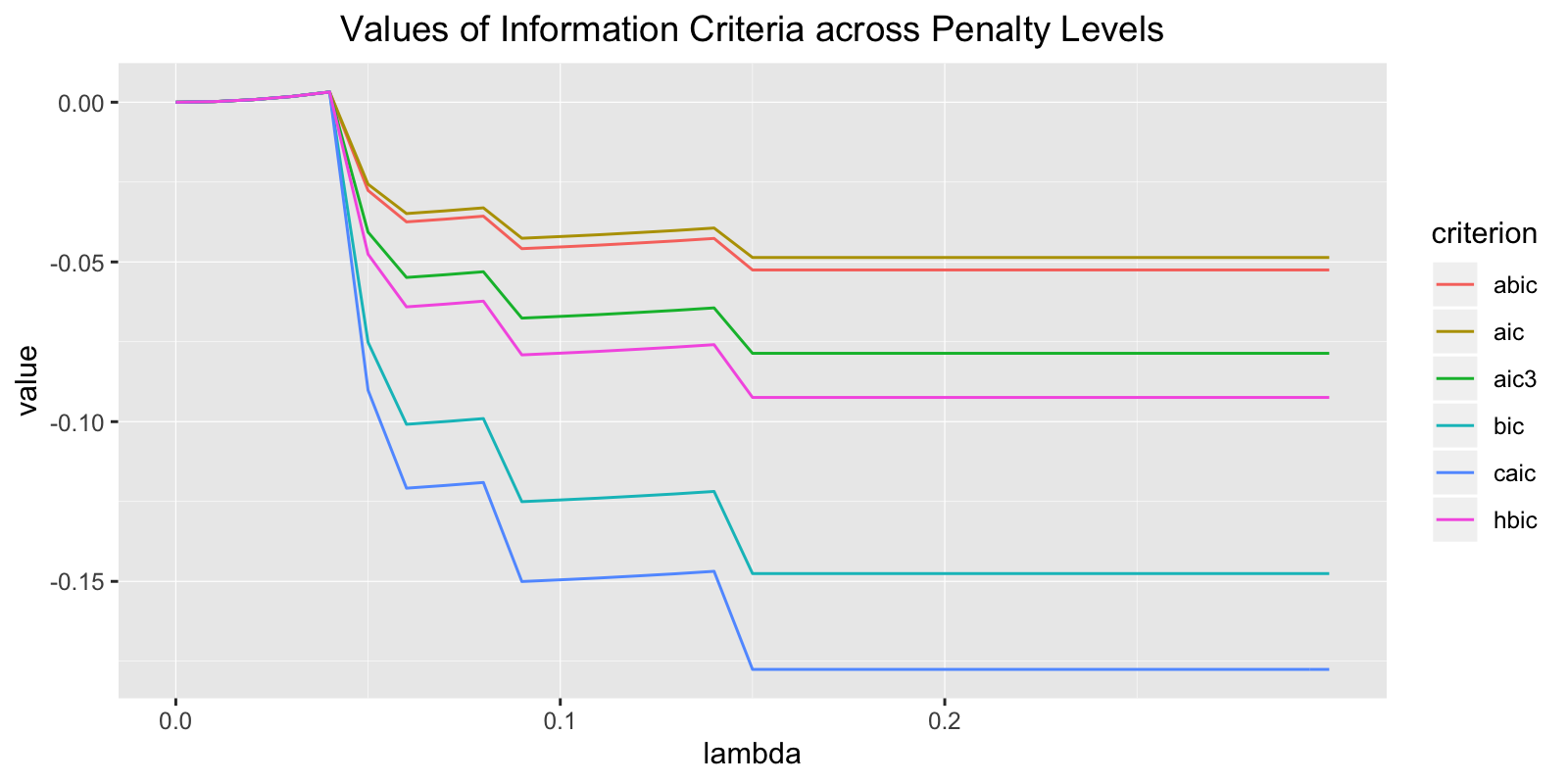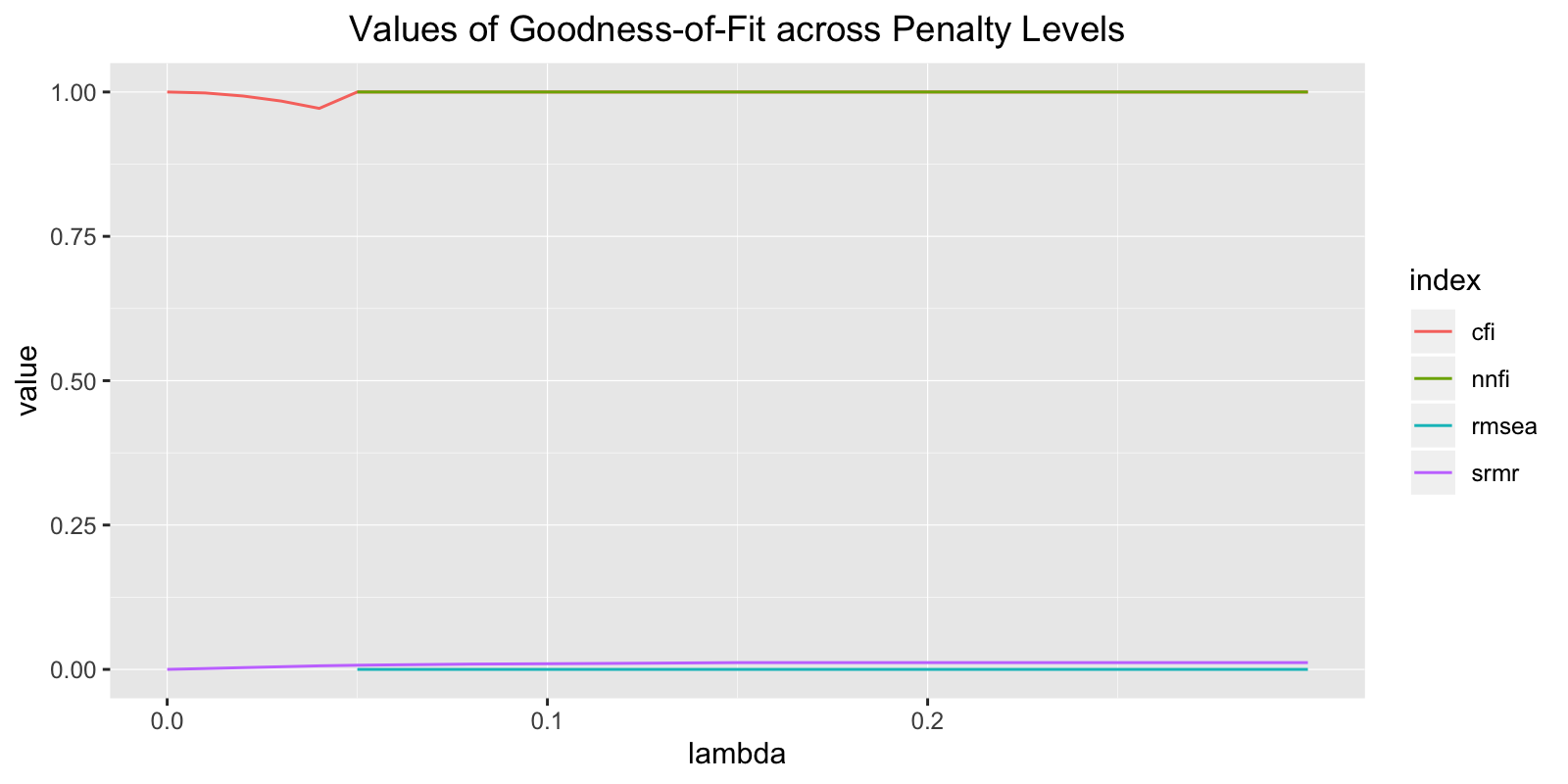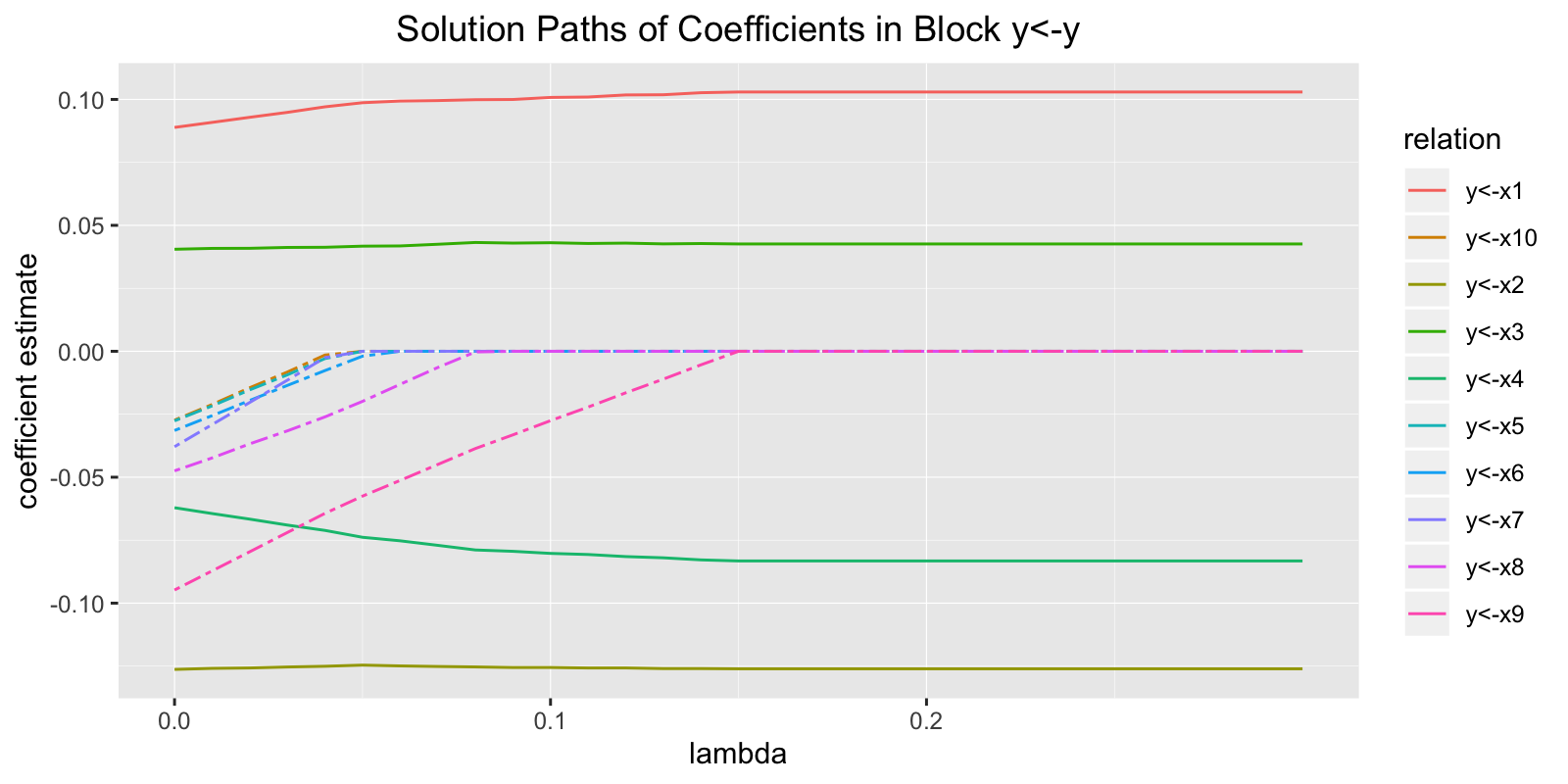# Regression Analysis with Lasso Penalty

In this example, we will show how to use lslx to conduct regression analysis with lasso penalty.

## Data Generation

The following code is used to generate data for regression analysis.

set.seed(9487)
x <- matrix(rnorm(2000), 200, 10)
colnames(x) <- paste0("x", 1:10)
y <- matrix(rnorm(200), 200, 1)
data_reg <- data.frame(y, x)

The data set contains 200 observation on 10 covariates (x1 - x10) and a response variable (y). By the construction of the data, the 10 covariates are not useful to predict the response. The data is stored in a data.frame named data_reg.

## Model Specification

Model specification in lslx is quite similar to that in lavaan. However, different operators and prefix are used to accommodate the presence of penalized parameters. In the following specification, y is predicted by x1 - x10.

model_reg <- "y <= x1 + x2 + x3 + x4
y <~ x5 + x6 + x7 + x8 + x9 + x10"

The operator <= means that the regression coefficients from the RHS variables to the LHS variables are freely estimated. On the other hand, the operator <~ means that the regression coefficients from the RHS variables to the LHS variables are estimated with penalty. Details of model syntax can be found in the section of Model Syntax via ?lslx. After version 0.6.3, lslx also support basic lavaan operators, including =~, ~, and ~~.

## Object Initialization

lslx is written as an R6 class. Every time we conduct analysis with lslx, an lslx object must be initialized. The following code initializes an lslx object named lslx_reg.

library(lslx)
lslx_reg <- lslx$new(model = model_reg, data = data_reg) An 'lslx' R6 class is initialized via 'data' argument. Response Variables: y x1 x2 x3 x4 x5 x6 x7 x8 x9 x10  Here, lslx is the object generator for lslx object and $new() is the build-in method of lslx to generate a new lslx object. The initialization of lslx requires users to specify a model for model specification (argument model) and a data set to be fitted (argument sample_data). The data set must contain all the observed variables specified in the given model. In is also possible to initialize an lslx object via importing sample moments (see vignette("structural-equation-modeling")).

## Model Fitting

After an lslx object is initialized, method $fit() can be used to fit the specified model into the given data. lslx_reg$fit(penalty_method = "lasso", lambda_grid = seq(.00, .30, .01))
CONGRATS: Algorithm converges under EVERY specified penalty level.
Specified Tolerance for Convergence: 0.001
Specified Maximal Number of Iterations: 100 

The fitting process requires users to specify the penalty method (argument penalty_method) and the considered penalty levels (argument lambda_grid). In this example, the lasso penalty is implemented on the lambda grid seq(.00, .30, .01). All the fitting result will be stored in the fitting field of lslx_reg.

## Model Summarizing

Unlike traditional SEM analysis, lslx fit the model into data under all the penalty levels considered. To summarize the fitting result, a selector to determine an optimal penalty level must be specified. Available selectors can be found in the section of Penalty Level Selection via ?lslx. The following code summarize the fitting result under the penalty level selected by Akaike information criterion (AIC).

lslx_reg$summarize(selector = "aic") General Information number of observations 200 number of complete observations 200 number of missing patterns none number of groups 1 number of responses 11 number of factors 0 number of free coefficients 71 number of penalized coefficients 6 Numerical Conditions selected lambda 0.300 selected delta none selected step none objective value 0.011 objective gradient absolute maximum 0.000 objective Hessian convexity 0.778 number of iterations 6.000 loss value 0.011 number of non-zero coefficients 71.000 degrees of freedom 6.000 robust degrees of freedom 5.734 scaling factor 0.956 Fit Indices root mean square error of approximation (rmsea) 0.000 comparative fit index (cfi) 1.000 non-normed fit index (nnfi) 1.000 standardized root mean of residual (srmr) 0.012 Likelihood Ratio Test statistic df p-value unadjusted 2.279 6.000 0.892 mean-adjusted 2.384 6.000 0.881 Root Mean Square Error of Approximation Test estimate lower upper unadjusted 0.000 0.000 0.057 mean-adjusted 0.000 0.000 0.058 Coefficient Test (Std.Error = "sandwich") Regression type estimate std.error z-value p-value lower upper y<-x1 free 0.103 0.071 1.452 0.073 -0.036 0.242 y<-x2 free -0.126 0.069 -1.831 0.034 -0.261 0.009 y<-x3 free 0.043 0.073 0.581 0.281 -0.101 0.186 y<-x4 free -0.083 0.072 -1.149 0.125 -0.225 0.059 y<-x5 pen 0.000 - - - - - y<-x6 pen 0.000 - - - - - y<-x7 pen 0.000 - - - - - y<-x8 pen 0.000 - - - - - y<-x9 pen 0.000 - - - - - y<-x10 pen 0.000 - - - - - Covariance type estimate std.error z-value p-value lower upper x2<->x1 free 0.101 0.085 1.192 0.117 -0.065 0.268 x3<->x1 free 0.071 0.074 0.956 0.170 -0.074 0.216 x4<->x1 free 0.168 0.082 2.046 0.020 0.007 0.328 x5<->x1 free 0.069 0.078 0.889 0.187 -0.083 0.221 x6<->x1 free -0.281 0.078 -3.578 0.000 -0.434 -0.127 x7<->x1 free -0.055 0.068 -0.806 0.210 -0.187 0.078 x8<->x1 free 0.083 0.076 1.083 0.139 -0.067 0.232 x9<->x1 free -0.082 0.075 -1.090 0.138 -0.230 0.066 x10<->x1 free -0.027 0.071 -0.379 0.353 -0.165 0.112 x3<->x2 free 0.042 0.070 0.605 0.273 -0.094 0.179 x4<->x2 free -0.011 0.076 -0.152 0.440 -0.160 0.137 x5<->x2 free 0.020 0.078 0.255 0.400 -0.132 0.172 x6<->x2 free -0.142 0.072 -1.972 0.024 -0.284 -0.001 x7<->x2 free 0.031 0.066 0.473 0.318 -0.098 0.161 x8<->x2 free 0.017 0.077 0.223 0.412 -0.134 0.169 x9<->x2 free 0.012 0.076 0.156 0.438 -0.138 0.162 x10<->x2 free -0.047 0.068 -0.693 0.244 -0.180 0.086 x4<->x3 free -0.051 0.072 -0.705 0.240 -0.191 0.090 x5<->x3 free -0.095 0.072 -1.319 0.094 -0.236 0.046 x6<->x3 free 0.082 0.080 1.029 0.152 -0.074 0.238 x7<->x3 free 0.073 0.071 1.025 0.153 -0.067 0.213 x8<->x3 free -0.120 0.071 -1.693 0.045 -0.259 0.019 x9<->x3 free 0.003 0.071 0.047 0.481 -0.136 0.142 x10<->x3 free -0.062 0.072 -0.856 0.196 -0.202 0.079 x5<->x4 free 0.078 0.074 1.052 0.146 -0.068 0.224 x6<->x4 free 0.015 0.076 0.201 0.420 -0.133 0.164 x7<->x4 free -0.054 0.065 -0.837 0.201 -0.180 0.072 x8<->x4 free 0.173 0.070 2.456 0.007 0.035 0.311 x9<->x4 free 0.101 0.070 1.455 0.073 -0.035 0.238 x10<->x4 free 0.027 0.075 0.358 0.360 -0.119 0.173 x6<->x5 free -0.100 0.073 -1.372 0.085 -0.242 0.043 x7<->x5 free -0.136 0.071 -1.908 0.028 -0.275 0.004 x8<->x5 free 0.126 0.074 1.690 0.046 -0.020 0.271 x9<->x5 free -0.061 0.079 -0.763 0.223 -0.216 0.095 x10<->x5 free 0.071 0.077 0.914 0.180 -0.081 0.222 x7<->x6 free 0.014 0.071 0.202 0.420 -0.125 0.153 x8<->x6 free 0.035 0.075 0.468 0.320 -0.112 0.182 x9<->x6 free 0.026 0.067 0.383 0.351 -0.106 0.157 x10<->x6 free -0.017 0.075 -0.224 0.411 -0.165 0.131 x8<->x7 free -0.082 0.064 -1.277 0.101 -0.208 0.044 x9<->x7 free -0.018 0.065 -0.274 0.392 -0.146 0.110 x10<->x7 free -0.096 0.060 -1.604 0.054 -0.213 0.021 x9<->x8 free -0.111 0.068 -1.638 0.051 -0.243 0.022 x10<->x8 free 0.156 0.071 2.189 0.014 0.016 0.296 x10<->x9 free -0.145 0.074 -1.948 0.026 -0.290 0.001 Variance type estimate std.error z-value p-value lower upper y<->y free 1.104 0.112 9.902 0.000 0.886 1.323 x1<->x1 free 1.206 0.116 10.429 0.000 0.980 1.433 x2<->x2 free 1.164 0.109 10.687 0.000 0.950 1.377 x3<->x3 free 1.035 0.094 10.958 0.000 0.850 1.220 x4<->x4 free 1.010 0.087 11.669 0.000 0.840 1.179 x5<->x5 free 1.078 0.113 9.578 0.000 0.858 1.299 x6<->x6 free 1.057 0.114 9.236 0.000 0.832 1.281 x7<->x7 free 0.839 0.078 10.794 0.000 0.687 0.992 x8<->x8 free 0.986 0.089 11.068 0.000 0.811 1.160 x9<->x9 free 1.022 0.112 9.108 0.000 0.802 1.241 x10<->x10 free 0.991 0.087 11.369 0.000 0.821 1.162 Intercept type estimate std.error z-value p-value lower upper y<-1 free -0.002 0.073 -0.034 0.487 -0.146 0.141 x1<-1 free -0.033 0.078 -0.424 0.336 -0.185 0.119 x2<-1 free -0.083 0.076 -1.083 0.139 -0.232 0.067 x3<-1 free 0.072 0.072 1.001 0.158 -0.069 0.213 x4<-1 free -0.025 0.071 -0.353 0.362 -0.164 0.114 x5<-1 free -0.050 0.073 -0.683 0.247 -0.194 0.094 x6<-1 free -0.096 0.073 -1.321 0.093 -0.238 0.046 x7<-1 free 0.027 0.065 0.414 0.339 -0.100 0.154 x8<-1 free 0.048 0.070 0.680 0.248 -0.090 0.185 x9<-1 free 0.001 0.071 0.016 0.494 -0.139 0.141 x10<-1 free 0.024 0.070 0.346 0.365 -0.114 0.162 In this example, we can observe that all of the penalized coefficients are identified as zero, which is consistent with their population values. The $summarize() method also shows the result of significance tests for the coefficients. In lslx, the default standard errors are calculated based on a sandwich formula whenever raw data is available. It is generally valid even when the model is misspecified and the data is not normal distributed. However, it may not be valid after selecting an optimal penalty level.

## Visualization

lslx provides four methods for visualizing the fitting results. The method $plot_numerical_condition() shows the numerical condition under all the penalty levels. The following code plots the values of n_iter_out (number of iterations in outer loop), objective_gradient_abs_max (maximum of absolute value of gradient of objective function), and objective_hessian_convexity (minimum of univariate approximate hessian). The plot can be used to evaluate the quality of numerical optimization. n_iter_out shows that the algorithm converges quickly under all the penalty levels. objective_gradient_abs_max and objective_hessian_convexity indicate that the obtained coefficients are valid minimizers under all the penalty levels. lslx_reg$plot_numerical_condition()The method $plot_information_criterion() shows the values of information criteria under all the penalty levels. The plot shows that an optimal value of lambda is any value larger than 0.15. lslx_reg$plot_information_criterion()The method $plot_fit_index() shows the values of fit indices under all the penalty levels. lslx_reg$plot_fit_index()
Warning: Removed 10 rows containing missing values (geom_path).The method $plot_coefficient() shows the solution path of coefficients in the given block. The following code plots the solution paths of all coefficients in the block y<-y, which contains all the regression coefficients from observed variables to observed variables. We can see that all the regression coefficients become zero when the value of lambda is larger than 0.15. lslx_reg$plot_coefficient(block = "y<-y")## Objects Extraction

In lslx, many quantities related to SEM can be extracted by extract-related method. For example, the coefficient under the penalty level selected by aic can be obtained by

lslx_reg$extract_coefficient(selector = "aic")  y<-1/g x1<-1/g x2<-1/g x3<-1/g x4<-1/g x5<-1/g x6<-1/g x7<-1/g -0.00246 -0.03294 -0.08263 0.07199 -0.02508 -0.05018 -0.09601 0.02681 x8<-1/g x9<-1/g x10<-1/g y<-x1/g y<-x2/g y<-x3/g y<-x4/g y<-x5/g 0.04775 0.00111 0.02436 0.10295 -0.12607 0.04261 -0.08325 0.00000 y<-x6/g y<-x7/g y<-x8/g y<-x9/g y<-x10/g y<->y/g x1<->x1/g x2<->x1/g 0.00000 0.00000 0.00000 0.00000 0.00000 1.10413 1.20623 0.10137 x3<->x1/g x4<->x1/g x5<->x1/g x6<->x1/g x7<->x1/g x8<->x1/g x9<->x1/g x10<->x1/g 0.07090 0.16763 0.06901 -0.28053 -0.05460 0.08263 -0.08223 -0.02676 x2<->x2/g x3<->x2/g x4<->x2/g x5<->x2/g x6<->x2/g x7<->x2/g x8<->x2/g x9<->x2/g 1.16395 0.04209 -0.01149 0.01977 -0.14231 0.03125 0.01724 0.01193 x10<->x2/g x3<->x3/g x4<->x3/g x5<->x3/g x6<->x3/g x7<->x3/g x8<->x3/g x9<->x3/g -0.04697 1.03474 -0.05058 -0.09476 0.08204 0.07300 -0.11996 0.00334 x10<->x3/g x4<->x4/g x5<->x4/g x6<->x4/g x7<->x4/g x8<->x4/g x9<->x4/g x10<->x4/g -0.06150 1.00961 0.07830 0.01525 -0.05398 0.17313 0.10138 0.02669 x5<->x5/g x6<->x5/g x7<->x5/g x8<->x5/g x9<->x5/g x10<->x5/g x6<->x6/g x7<->x6/g 1.07833 -0.09960 -0.13574 0.12568 -0.06061 0.07054 1.05658 0.01436 x8<->x6/g x9<->x6/g x10<->x6/g x7<->x7/g x8<->x7/g x9<->x7/g x10<->x7/g x8<->x8/g 0.03514 0.02572 -0.01691 0.83915 -0.08207 -0.01792 -0.09597 0.98588 x9<->x8/g x10<->x8/g x9<->x9/g x10<->x9/g x10<->x10/g -0.11069 0.15616 1.02161 -0.14454 0.99144  Here, /g means the coefficient belongs to the group g which is default group name. We may also check the quality of optimization by viewing the subgradient of objective function lslx_reg$extract_objective_gradient(selector = "aic", type = "effective")
                 [,1]
y<-1/g      -5.27e-06
x1<-1/g      0.00e+00
x2<-1/g      0.00e+00
x3<-1/g      2.65e-23
x4<-1/g     -5.29e-23
x5<-1/g     -5.54e-24
x6<-1/g      0.00e+00
x7<-1/g      0.00e+00
x8<-1/g     -3.99e-23
x9<-1/g     -8.87e-24
x10<-1/g    -4.43e-24
y<-x1/g      2.98e-05
y<-x2/g      1.18e-04
y<-x3/g      1.37e-05
y<-x4/g      1.57e-04
y<->y/g      9.45e-06
x1<->x1/g    3.69e-18
x2<->x1/g   -2.88e-18
x3<->x1/g    2.06e-18
x4<->x1/g    9.54e-18
x5<->x1/g   -3.47e-18
x6<->x1/g   -1.30e-17
x7<->x1/g    1.65e-17
x8<->x1/g    1.73e-18
x9<->x1/g   -3.47e-18
x10<->x1/g   0.00e+00
x2<->x2/g   -3.55e-18
x3<->x2/g    3.58e-18
x4<->x2/g   -1.13e-17
x5<->x2/g    4.34e-18
x6<->x2/g   -1.56e-17
x7<->x2/g   -1.73e-18
x8<->x2/g    1.21e-17
x9<->x2/g    1.73e-17
x10<->x2/g   0.00e+00
x3<->x3/g    7.47e-18
x4<->x3/g    1.73e-17
x5<->x3/g    6.07e-18
x6<->x3/g   -2.13e-17
x7<->x3/g   -2.43e-17
x8<->x3/g   -1.08e-17
x9<->x3/g   -2.08e-17
x10<->x3/g  -7.37e-18
x4<->x4/g   -2.93e-17
x5<->x4/g   -4.34e-18
x6<->x4/g   -8.67e-18
x7<->x4/g    8.67e-18
x8<->x4/g    6.85e-17
x9<->x4/g    2.78e-17
x10<->x4/g   1.73e-18
x5<->x5/g    2.03e-17
x6<->x5/g    6.23e-18
x7<->x5/g   -5.51e-18
x8<->x5/g    8.81e-18
x9<->x5/g    1.96e-18
x10<->x5/g  -9.98e-19
x6<->x6/g   -5.71e-18
x7<->x6/g    5.51e-18
x8<->x6/g    7.03e-18
x9<->x6/g   -3.98e-18
x10<->x6/g   1.36e-17
x7<->x7/g    3.41e-17
x8<->x7/g   -8.55e-18
x9<->x7/g    1.83e-17
x10<->x7/g   1.63e-17
x8<->x8/g   -3.43e-17
x9<->x8/g   -1.93e-17
x10<->x8/g   6.20e-18
x9<->x9/g   -2.68e-18
x10<->x9/g  -8.24e-18
x10<->x10/g  1.01e-18

Here, the type argument is used to specify which types of parameters are used to calculate related quantities. type = "effective" indicates that only freely estimated and penalized non-zero parameters are used. By default, type = "all". The subgradient shows that the obtained solution is optimal since all the elements are very small.